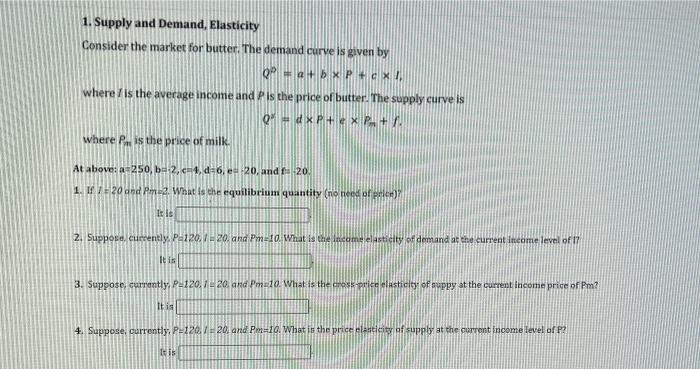### Create an Account

Already have account?

### Forgot Your Password ?

Home / Questions / Supply and Demand, Elasticity Consider the market for butter. The demand curve is given by...

# Supply and Demand, Elasticity Consider the market for butter. The demand curve is given by Pla+bxp + ex!! where is the average income and Pis the price of butter. The supply curve is Q dx P+ X P

Supply and Demand, Elasticity Consider the market for butter. The demand curve is given by Pla+bxp + ex!! where is the average income and Pis the price of butter. The supply curve is Q dx P+ X P. where is the price of milk At above: 250,b=2, 4, 6, e 20 and 20 1. If 720 and Pm2. What is the equilibrium quantity (no need of pele) It is 2. Suppose, currently P220./20. and Pm10. What is the income elasticity of demand at the current ineome level of 17 It is 3. Suppose. currently, P-120, 120, and melo What is the most price elasticity of suppy at the current income price of Pm? It is 4. Suppose, currently, P-120, 7-20, and Pm=10. What is the price elasticity of supply at the current income level of P?Apr 16 2021 View more View Less

#### Answer (Solved)Subscribe To Get Solution